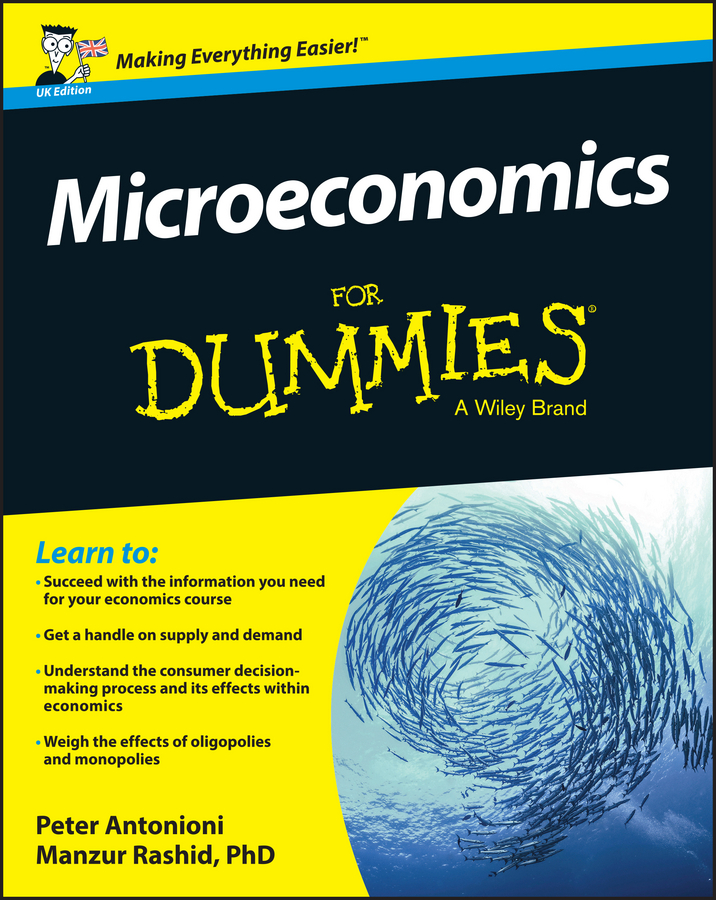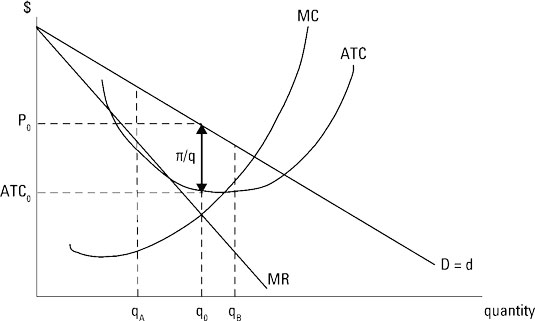##### Microeconomics For Dummies - UKQuite often it’s easier to determine the profit-maximizing quantity of output by focusing on the last unit you produce, or the marginal unit. In order to add to your profit, an additional or marginal unit of the good must add more to your revenue than it adds to your cost. In other words, marginal revenue is greater than marginal cost.

Stop at this output level because if you go beyond this point and continue to produce more, marginal cost is greater than marginal revenue — so you’re adding more to your cost than you’re adding to your revenue, and your total profit is decreasing.

In monopoly, only one firm is producing the good. Therefore, any customer who wants to buy the good must buy it from the monopoly. Thus, the demand for the firm’s product is the market demand.

In the illustration, the monopolist’s downward-sloping demand curve d is the same as the market demand curve D, or D = d. Given the linear demand curve, the marginal revenue curve has the same intercept on the vertical axis and is twice as steep as the demand curve. Marginal revenue is represented by the curve labeled MR.Marginal cost, MC, is upward-sloping and passes through the minimum point on average total cost, ATC.

To maximize profits, you produce the output level associated with marginal revenue equals marginal cost, or the output level q0 that corresponds to the point where the marginal revenue and marginal cost curves intersect.

Marginal revenue equals marginal cost maximizes total profit.

To find the price you charge, go from the profit-maximizing quantity of output, up to the demand curve and across. The profit-maximizing price corresponds to P0.

The fact that price is determined by the point where q0 hits the demand curve emphasizes the constraint that market demand places on the monopolist’s ability to set price. If advertising increases demand — shifts the demand curve to the right — you’re able to set a higher price.

If you produce less than q0 output, for example qA, those units still add more to your revenue than they add to your cost. For those units, marginal revenue is greater than marginal cost. You increase profits by producing more output moving toward q0.

If you produce output beyond q0, such as qB, those units add more to your cost than they add to your revenue because marginal cost is greater than marginal revenue. These units reduce your profit so you should cut back production to q0.

As is the case for any firm, a monopolist determines profit per unit by subtracting average total cost from price. In the illustration, profit per unit is represented by the double-headed arrow labeled π/q. Total profit is determined by multiplying profit per unit by the number of units sold, q0.